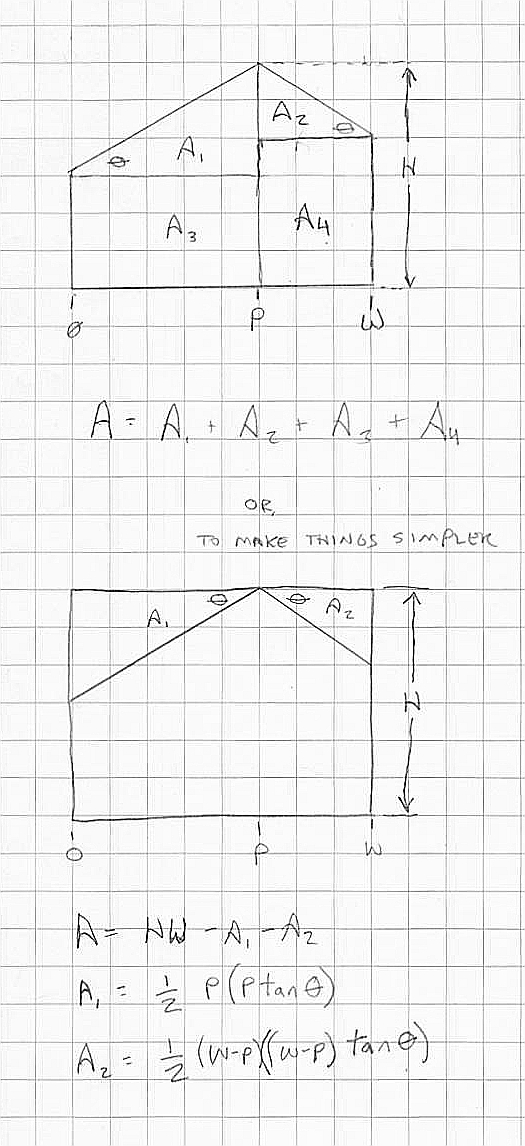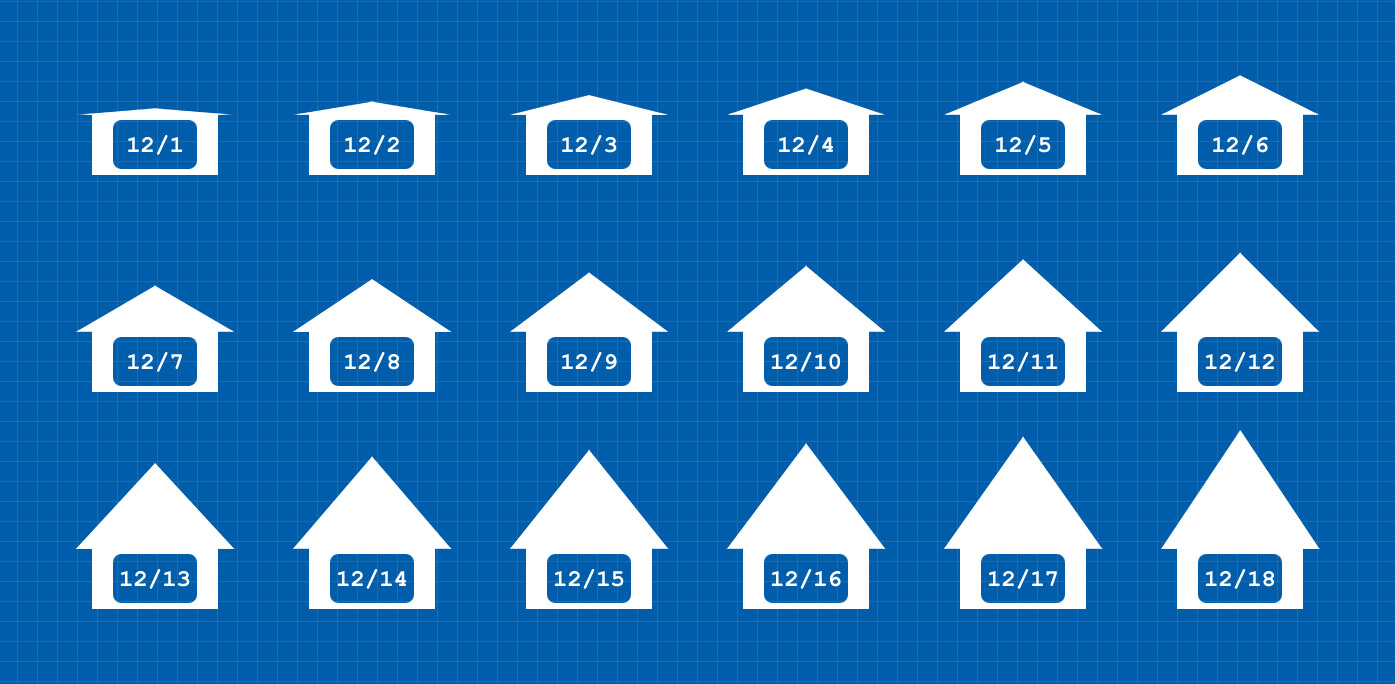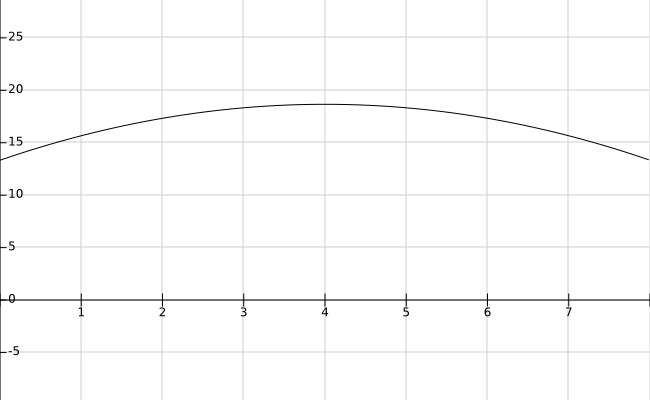# Roof design and loft space: doing the math

From almost the beginning of my tiny house design, I'd wanted a roof with an off-center ridge (AKA "saltbox", but I didn't learn the term until recently).  My thinking was that I would get the same amount of usable head space as having the ridge in the center, but would have it in a more desirable location, i.e. near where your head would be when sleeping. But the other day I decided to actually check and see if this assumption was true, and I was, frankly, a little surprised*: for a peaked roof, the most space** is obtained when the peak is in the middle, and the least when a shed roof is used.loft space crosssections

Okay, so you're probably thinking, "what the hell dude, that doesn't help". Actually it does.  The slope of your roof is θ.  Obviously, you get your maximum space (cross section area) when θ = 0 (flat roof).  A flat roof isn't ideal for most climates (it doesn't naturally shed water), so most people choose to have some slope to their roof. Traditional roof slopes are given in the form of a ratio, say 4:12 (4 in 12).  That means that the roof rises 4 feet for every 12 feet horizontal. Recall from trig that the tangent of an angle is the ratio of rise over run, so 4:12 is equivalent to an angle of 18.4°.  Here is an image from the wikipedia page on roof pitch to help visualize thisExample of roof pitches

The first thing you want to do if you are trying to maximize the loft space in your design is decide on what the minimum roof slope that works for your climate and aesthetics.  For my tiny house, I chose approximately 8:12*** for the main roof and 4:12 for the shed dormer.  Personally, I like the look of a steeper roof, and I was willing to go with less overall space to get the look I wanted. For my region (Michigan) 3:12 is a good minimum because of snow loads (but because tiny houses aren't very wide you can get away with a much shallower roof.)

Looking at the equation for area, how do we use this to maximize our loft space? Calculus, of course! Since we've chosen the slope of our roof based on other criteria, and the width and height are constrained, the only variable is P, so loft space (volume really, but we're only looking at the cross section) is a function of P, the distance of the roof ridge from the wall.

$A=f(P)=HW - \frac{tan\theta}{2}(W^2 -2WP +2P^2)$

If we graph this (approximately, using some reasonable values), we see that the area has a maximum value for some value of P.Cross sectional area of the loft is vertical, distance P from the wall is the horizontal; H is 3, W is 8, pitch is 4:12

We can find that point by taking the derivative of $$f(P)$$ and setting it equal to 0.

$f(P)=HW - \frac{tan\theta}{2}(W^2 -2WP +2P^2)$

$f'(P)=- \frac{tan\theta}{2}( -2W +4P) =0$

$2W-4P=0$

$P=\frac{1}{2} W$

An example makes it easier to understand.  Let's assume that our loft is 8' wide, and the peak of the roof is 4' above the loft. Let's also assume we chose a 4:12 pitch (18.4°). With a gable roof, the roof height at the walls will be 16" below the peak, or 2' 8.  That's at each wall.  If instead we use a shed roof, the roof peak (4') will be at one wall, the height in the center will be 2' 8, and the height at the other wall will be a meager 1' 4.

So, after doing the math --which is what engineers do-- it is clear that we can maximize loft space by first choosing a low slope roof, then using a gabled (ridge in the middle) roof. This is not intended as a definitive "hell no" to shed roofs. They have some advantages: they're easy to frame and they look modern. But if head height in the loft is a concern, skip the shed

* I shouldn't have been surprised, but I hadn't given it any thought, and I don't have good math intuition. A lot of people don't.

** "Most space", but not definitively most useable space.  Any roof where you have adequate headroom near the walls has the same useful space. A low-slope shed or saltbox-that-isn't-too-off-center roof can provide the functionally equivalent amount of loft space.

*** Although I wrote that I chose 8:12, this ratio was actually the result of a decision to use untrimmed 8' 2x4s for my side walls.  Add the bottom and top plates, and the height of the trailer frame, and my exterior wall height was set; the peak height is limited by law to 13' 6 (I used 13' 5 to leave room for roofing material); so my roof pitch was the result of other decisions.  I could have made it shallower or steeper, but it would have cost more in time and materials.